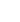15min:
MILLIMETER WAVE SPECTRA OF THE INTERNAL ROTATION EXCITED STATES OF (o)H2-H2O AND (o)H2-D2O.

K. HARADA, Y. IWASAKI, T. GIESEN AND K. TANAKA, Department of Chemistry, Faculty of Science, Kyushu University, Hakozaki, Higashiku, Fukuoka, 812-8581 JAPAN.

~~~~H2-H2O is a weakly bound complex and it has a various states according to the internal rotation for both H2 and H2O moieties. In our previous study, we have reported the pure rotational transitions of the (o)H2 complex in the ground H2O rotational state, 000(), for both H2-H2O and H2-D2O, where (o)H2 (j H2 =1) is rotating perpendicular to the intermolecular axis to give the projection of j H2 to the axis k H2 to be zero (i.e.state).

~~~~In the present study, we have observed the rotational transitions for the 000 () states in the millimeter-wave region up to 220 GHz, where the (o)H2 is rotating around the intermolecular axis to give the projection k H2 to be one (i.e.state). The center of mass bond lengths derived from the observed rotational constants for 000 () are longer by 5 % than those for 000 (), while force constants for the intermolecular stretching for 000 () derived from centrifugal distortion constants are smaller by 23 % than those for 000 (), suggesting theandsubstates have quite different structures.

~~~~The recent theoretical calculation indicates that for 000(), (o)H2 is bound to the oxygen site of H2O, while for the 000 () state, (o)H2 to the hydrogen site of H2O, and the 000() state is by 14 cm-1 more stable than the 000 () state. Observed molecular constants for 000() and () are consistent with the structures given by the theoretical calculation. ~~~~We also observed the rotational spectrum in the 101 () and () states, whereandcorrespond to the rotation of H2O perpendicular and parallel to the intermolecular axis and (o)H2 is calculated to be bound to the oxygen site of H2O. The energy difference between the 101 () and () states will be discussed due to the Criolis interaction between these substates.